• data.csv中包含了一个新闻标题列表，试通过近似检测方法，通过Jaccard相似度，检测相似文章，将结果保存到csv文件中，不同文章间用空行隔开。 Work 思路： 两个词作为一段来计算，末尾不够截掉 Jaccard相关系数大于...
• 文章目录Jaccard相似度介绍Jaccard相似度计算推荐结果代码及实现基本介绍Jaccard相似度计算代码协同过滤推荐代码实现 Jaccard相似度介绍 两个集合的交集元素个数在并集中所占的比例, 非常适用于布尔向量表示 分子是...


文章目录
Jaccard相似度介绍Jaccard相似度计算推荐结果代码及实现基本介绍Jaccard相似度计算代码协同过滤推荐代码实现

Jaccard相似度介绍
两个集合的交集元素个数在并集中所占的比例, 非常适用于布尔向量表示分子是两个布尔向量做点积计算, 得到的就是交集元素的个数分母是两个布尔向量做或运算, 再求元素和杰卡德相似度适用于隐式反馈数据(0,1布尔值)，主要应用于是否收藏,是否点击,是否加购物车。
简单地来说就是交集/并集 J(A,B)=|A∩B|/|A∪B|
Jaccard相似度计算推荐结果代码及实现
基本介绍
我们通过简单的一个DataFrame例子来实现其算法，最终输出结果。通常有两种协同过滤：基于用户的协同过滤推荐（User-based CF）、基于物品的协同过滤推荐（Item-based CF）。这里我们会构建一个用户+物品的DataFrame。  这里为了方便，可以使用jupyternotebook进行书写
import numpy as np
import pandas as pd

users = ["Thomas", "Cauchy", "Alice", "Bob", "Alex"]
items = ["iPad", "MacBook", "iPhone", "iWatch", "AirPods"]
#用户购买记录数据集
datasets = [
[1,0,1,1,0],
[1,0,0,1,1],
[1,0,1,0,0],
[0,1,0,1,1],
[1,1,1,0,1],
]

df = pd.DataFrame(datasets,
columns=items,
index=users)
print(df)
print(df.index)
print(df.columns)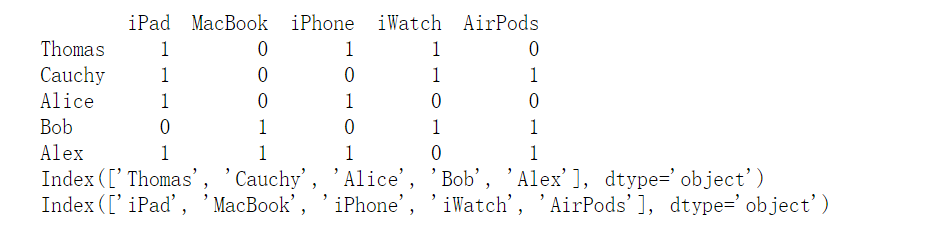Jaccard相似度计算代码
#导入杰卡德相似度
from sklearn.metrics import jaccard_score
#结果
0.2

#计算所有的数据两两的杰卡德相似系数
from sklearn.metrics.pairwise import pairwise_distances
#计算所有的数据两两的杰卡德相似系数（1-jaccard距离就是相似度）
user_similar = 1-pairwise_distances(df.values,metric='jaccard')
user_similar = pd.DataFrame(user_similar,columns=users,index = users)
#用户相似度
user_similar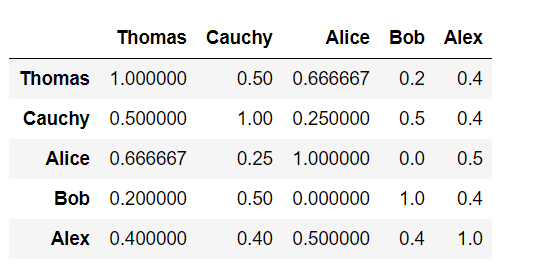#以相同的思路我们来试着做一下物品的相似度（记住物品需要先进行转置才可以计算）
item_similar = 1-pairwise_distances(df.T.values,metric='jaccard')
item_similar = pd.DataFrame(item_similar,columns=items,index = items)
item_similar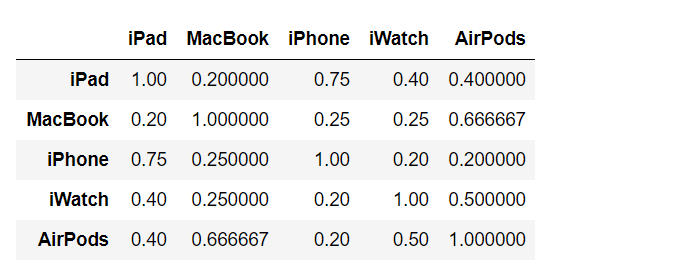协同过滤推荐代码实现
通过计算杰卡德相似度，已经得出了用户相似度，接下来我们就需要进行协同过滤推荐了。实现协同过滤推荐有2个步骤：
找出最相似的人或物品：TOP-N相似的人或物品
#为每一个用户找到最相似的2个用户

#创建空的dict保存最终结果
topN_users = {}
#遍历每一行数据
for i in user_similar.index:
#取出每一列数据， 删除自己，按照相似度排序（降序）
_df = user_similar.loc[i].drop([i])
_df_sorted = _df.sort_values(ascending = False)
#排序之后切片取出前两个值，传入空dict
top2 = list(_df_sorted.index[:2])
topN_users[i] = top2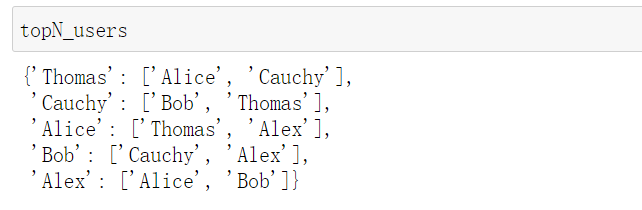根据相似的人或物品产生推荐结果
#根据topn的相似用户构建推荐结果
rs_results={}
for user,sim_users in topN_users.items():
rs_result = set() #为每一用户保存推荐结果
for sim_user in sim_users:
#将0替换为控制，去掉空值，做union联合
rs_result = rs_result.union(set(df.loc[sim_user].replace(0,np.nan).dropna().index))

#过滤掉已经购买的商品（得到的结果需要减去user已经购买过的）
rs_result -= set(df.loc[user].replace(0,np.nan).dropna().index)
#将结果传入到dict中
rs_results[user] = rs_result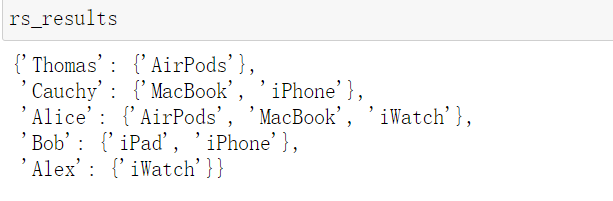展开全文算法 python 推荐系统 协同过滤
• uids = set(uids) # uids是list，转为set jaccard_sims = uid_sets.apply(lambda x: jaccard_similarity(x, uids)).tolist() #计算每个日记的uid和当前uid的计算相似度 recs = (i for i in zip(group_ids, jaccard_...
from pyspark.sql import SparkSession, functions as F
import heapq
from pyspark.sql.types import StructType, StructField, LongType,FloatType, ArrayType, IntegerType

spark = SparkSession.builder.appName("search_statistics").getOrCreate()
print("现有的dataframe结构")
log.printSchema()
log.show(3)
log = log.withColumn("uid", log["uid"].cast(IntegerType())).withColumn("target_id", log["target_id"].cast(IntegerType())).withColumn("device_id", log["device_id"].cast(IntegerType()))
print("格式转换后的dataframe")
log.printSchema()
print("去重前size %d" % log.count())
log = log.selectExpr('uid', 'target_id group_id', 'device_id').distinct() #去重
print("去重后size %d" % log.count())
log.show()

df = log.join(bad_device, 'device_id', 'left_anti').filter('uid!=0') #使用left_anti对黑名单数据进行剔除。同时剔除uid=0
print("log大小 %d  df大小 %d" % (log.count(), df.count()))

# df =df.select(F.when(F.col('uid') == 0, F.col('device_id') * -1).otherwise(F.col('uid')).alias('uid'), 'group_id') #对于uid等于0的，采取-device_id作为uid

df = df.groupBy('group_id').agg(F.collect_set('uid').alias('uids')).filter('size(uids) > 5') #把每个group_id的uid聚合成set。并剔除浏览用户小于5个的日记（数据太小，不利于算相似日记）
df.show()

pdf = df.select('group_id', 'uids').toPandas() #sparkDF转pandas数据，方便本地计算
group_ids = [int(i) for i in pdf['group_id'].tolist()]
uid_sets = pdf['uids'].apply(lambda x: set(x))

#  自定义udf返回类型
TOP_N_TYPE = StructType([
StructField('group_id', LongType()),
StructField("score", FloatType()),
])
def jaccard_similarity(a, b):
a_len = len(a)
b_len = len(b)
if a_len == 0 and b_len == 0:
return 0
else:
return len(a & b) / len(a | b)
# return len(a & b) / sqrt(a_len * b_len)
def item_recommend(group_ids, uid_sets, n_rec, group_id, uids):
"""相关的topN帖子推荐
:param group_ids: {list} 所有的group_id，用于将group_id和杰卡得相似度关联 n个group_id
:param uid_sets: {pandas.core.series.Series} 所有帖子的所有浏览用户集合  n行用户id的set 和group_id顺序对应
:param n_rec: {int} 保留n个最相关的帖子
:param uids: {list} 当前帖子的浏览用户id集合
:param group_id: {list} 当前帖子id，用于在相关贴子中去除当前帖子
:return: {list} [(post_id, js_similarity),...]
"""
uids = set(uids)  # uids是list，转为set
jaccard_sims = uid_sets.apply(lambda x: jaccard_similarity(x, uids)).tolist() #计算每个日记的uid和当前uid的计算相似度
recs = (i for i in zip(group_ids, jaccard_sims) if i != group_id)
return heapq.nlargest(n_rec, recs, key=lambda x: x) #堆排序取Top100

n_rec =100 #每个日记推荐最相似的100个日记
jaccard_item_rec = F.udf(lambda group_id, uids:item_recommend(group_ids, uid_sets, n_rec, group_id, uids), ArrayType(TOP_N_TYPE)) #js_item_rec就是一个注册后的udf函数
"""冒号左侧是udf的输入参数，右侧是uid对应的函数"""

df_sim = df.select('group_id',jaccard_item_rec('group_id', 'uids').alias('recs'))
df_sim.show()

表：一个是item的浏览日志表。还要一个作弊用户id表。
展开全文• 简单介绍一下Jaccard系数:广义Jaccard系数可以用于文档数据，并在二元属性情况下归约为Jaccard系数。...其公式与余弦相似度计算有点相似   http://hi.baidu.com/soulingm/blog/item/28ff4
简单介绍一下Jaccard系数:广义Jaccard系数可以用于文档数据，并在二元属性情况下归约为Jaccard系数。广义Jaccard系数又称Tanimoto系数。（有另一种系数也称为Tanimoto系数）。该系数用EJ表示，由下式定义： EJ(x,y)=(x*y)/(||x|*||x||+||y||*||y||-x*y) 其公式与余弦相似度的计算有点相似

http://hi.baidu.com/soulingm/blog/item/28ff41f88f43586a024f56d4.html

http://www.cnblogs.com/TtTiCk/archive/2007/07/09/811644.html
展开全文文档
• 1. 狭义Jaccard相似度，计算两个集合之间的相似程度，元素的“取值”为0或1 ...对集合A和B，Jaccard相似度计算如下： Jaccard（A, B）= |A intersect B| / |A union B| 相似度数值在[0, 1]之间，当A==
转自：http://blog.csdn.net/xceman1997/article/details/8600277
1. 狭义Jaccard相似度，计算两个集合之间的相似程度，元素的“取值”为0或1

对集合A和B，Jaccard相似度计算如下：

Jaccard（A, B）= |A intersect B| / |A union B|

相似度数值在[0, 1]之间，当A==B的时候，为1. 优缺点，就是元素的取值只能是0或者1，无法利用更丰富的信息。

由相似度，可以转换成Jaccard距离：

Jaccard distance （A, B） = 1 - Jaccard（A, B）

2. 广义Jaccard相似度，元素的取值可以是实数。又称为Tanimoto系数，用EJ来表示，计算方式如下：

EJ(A,B)=(A*B)/(||A||^2+||B||^2-A*B)

其中A、B分别表示为两个向量，集合中每个元素表示为向量中的一个维度，在每个维度上，取值通常是[0, 1]之间的值，A*B表示向量乘积，||A||^2表示向量的模，即 ||A||^2 = sqrt (a1^2 + a2^2 + a3^2 + ......)。

广义Jaccard相似度计算公式中，如果把分母的A*B去掉，并将||A||^2+||B||^2替换为（||A||^2）*（||B||^2），就转成了余弦相似度（cosine similarity）。

EJ中每个分量的取值可以是实数，通常在[0, 1]之间。对于两篇文档，分词之后，形成两个“词语--词频向量”，词语可以做为EJ的维度，如何将词频转换为实数值。借鉴tf/idf的思路。对于每个词语，有两个频度：1.在当前文档中的频度；2. 在所有文档中的频度。其中1相当于tf，与权重正相关；2相当于df，与权重反相关。

对于2，计算权重为

idf (w) = log (TotalWC/C(w))

C(w)是词语w在所有文档中出现的次数，TotalWC是所有文档中所有词的总词频。

对于1，权重就可以取词频本身 tf(w) = D(w)，D(w)表示在当前文档中w出现的次数。

具体计算的代码可以参考 “
http://www.cnblogs.com/TtTiCk/archive/2007/08/04/842819.html”的Documents.cs中的“SimilitudeValueToDocumentUsingGeneralizedJaccardCoefficient”函数。

3. 其他扩展方法

文章“
http://www.docin.com/p-461291267.html”给出了一种扩展方法，用最大最小值函数来代替乘积和模计算，如下：

EJ(A,B) = sum ( min(a1, b1) + min (a2, b2)... ) / sum ( max(a1, b1) + max (a2, b2).. )

即用向量中每个分量的的最小值和最大值来参与计算。

个人理解，这个可以做如下解释。当集合A中的元素a1出现C(a1)次的时候，我们可以认为集合中的元素是允许重复存在的，即集合A中有C(a1)个元素；集合B也是这样，有C(b1)个相同的元素，则A和B在这个元素上的交集就是min(a1, b1) ，并集就是max(a1, b1) ，这样上述公式就是利用狭义Jaccard相似度计算的结果。

参考文献：

wiki：http://en.wikipedia.org/wiki/Jaccard_similarity

http://blog.csdn.net/knzeus/article/details/6319137

http://www.cnblogs.com/TtTiCk/archive/2007/08/04/842819.html 有代码示例

http://www.cnblogs.com/live41/archive/2010/01/03/1638222.html

http://www.docin.com/p-461291267.html


展开全文• 对集合A和B，Jaccard相似度计算如下： Jaccard（A, B）= |A intersect B| / |A union B| 相似度数值在[0, 1]之间，当A==B的时候，为1. 优缺点，就是元素的取值只能是0或者1，无法利用更丰富的信息。 由...
• 两个节点a和b Jaccard相似度定义为： J(a, b) = (N(a) ∩ N(b)) / (N(a) ∪ N(b)) 其中N(a)是节点a的邻居的集合。 该模块允许您计算图中每个节点的相似度。 用法 var createCalculator = require('ngraph.jaccard...JavaScript
• 什么是jaccard相似度2. 计算方法2.1 二维2.2 推广到多维3. 推荐中的余弦相似度 1. 什么是jaccard相似度 交并比，交集比并集，表示多样性或者 2. 计算方法 2.1 二维 a⋅b=∥a∥∗∥b∥∗cos⁡θa \cdot b= \| a \| *...
• ## Jaccard相似度

千次阅读 2020-08-27 11:05:24
Jaccard相似度 杰卡德系数(Jaccard Index)，也称Jaccard相似系数(Jaccard similarity coefficient)，用于比较有限样本集之间的相似性与差异性。如集合间的相似性、字符串相似性、目标检测的相似性、文档查重等。...
• 输入任意两个字符串，根据公式来计算两个字符串的Jaccard相似度Jaccard相似度的公式为：import java.util.HashSet; import java.util.Scanner; import java.util.Set; public class StrJaccard { public static ...java
• Jaccard相似度的python实现； #import numpy as np #from scipy.spatial.distance import pdist#直接调包可以计算JC值 :需要两个句子长度一样；所以暂时不用 import jieba def Jaccrad(model, reference):#...
• J ( A , B ) = A ∩ B A ∪ B J(A,B) = \frac{A \cap B }{A \cup B} J(A,B)=A∪BA∩B​ 文本jaccard相似度python实现 计算两个文本的jaccard相似度，看着比较简单，把字符串拆分为两个集合，再分别球交集和并集即可...python
• 我研究了naive的不加权的余弦相似度和Jaccard相似度 老师要求我们研究他们的算法，由于我算法太渣，知道运算原理但是总结不出算法 请哪位大神能够指点一下，告诉我这两个算法的伪代码怎么弄，谢啦nlp 余弦相似 算法
• 目录 1、原生态Jaccard 1.1定义 1.2引申-Jaccard距离 1.3应用 2、语义版Jaccard ...在做自然语言处理的过程中，文本相似在有很重要的应用，我们经常会遇到...本节介绍 基于Jaccard相似度来进行语义相似度计算。 ...java 算法 机器学习 自然语言处理...